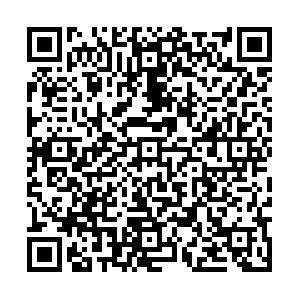## 留言板引用本文: 李雅芝，刘利利. 登革热传播模型的最优综合控制研究 [J]. 应用数学和力学，2022，43（4）：445-452LI Yazhi, LIU Lili. Study of the Optimal Integrated Control of a Dengue Transmission Model[J]. Applied Mathematics and Mechanics, 2022, 43(4): 445-452. doi: 10.21656/1000-0887.420258
 Citation: LI Yazhi, LIU Lili. Study of the Optimal Integrated Control of a Dengue Transmission Model[J]. Applied Mathematics and Mechanics, 2022, 43(4): 445-452.• 中图分类号: O193

## Study of the Optimal Integrated Control of a Dengue Transmission Model

• 摘要:

建立了一个登革热在蚊子和人之间传播的模型，引入了Wolbachia、自我保护和杀虫剂三种控制措施，分别从常数控制和时变控制两个方面进行探讨。首先，分析了常数控制对模型基本再生数的影响，研究发现：Wolbachia有助于减小基本再生数，且基本再生数与自我保护和杀虫剂呈负相关。其次，以使得感染数最少且实施成本最低为目标，使用Pontryagin极值原理讨论最优控制。最后，通过数值模拟展示了最优控制的效果。

• 图  1  常数控制对登革热感染人数的影响

Figure  1.  Effects of constant control on the number of dengue infections

图  2  控制策略对登革热感染人数的影响

Figure  2.  Effects of the control strategy on the number of dengue infections

图  3  最优综合控制与单一控制的比较：(a) 两种控制策略下$u_{1}$的变化图；(b) 两种控制策略下$u_{2}$的变化图

Figure  3.  Comparison between the optimal integrated control and the single control: (a) the variation diagram of $u_{1}$under 2 control strategies; (b) the variation diagram of $u_{2}$under 2 control strategies

4  不同权重对控制变量的影响：(a) 改变$B_{1}$$u_1$的影响，$A=1, B_{2}=1$；(b) 改变$B_{2}$$u_2$的影响，$A=1, B_{1}=1$；(c) A=1时，$u_{1}, u_{2}$的变化图，$B_{1}=1, B_{2}=1$；(d) $A=5$时，$u_{1}, u_{2}$的变化图，$B_{1}=1, B_{2}=1$4.  The influences of different weights on control variables: (a) the influence of $B_{1}$on $u_{1}$, $A=1, B_{2}=1$; (b) the influence of $B_{2}$on $u_{2}$, $A=1, B_{1}=1$; (c) the variation diagram of $u_{1}\; {\rm{and}} \;u_{2},$$A=1, B_{1}=1, B_{2}=1$; (d) the variation diagram of $u_{1}\; {\rm{and}} \;u_{2},$$A=5$, $B_{1}=1, B_{2}=1$表  1  模型(1)中各状态变量的含义

Table  1.   The meanings of variables in model (1)

 state variable biological meaning $S_{{\rm{mi}}}$ mosquito population infected with W and susceptible to D $S_{{\rm{mu}}}$ mosquito population not infected with W and susceptible to D $E_{{\rm{mi}}}$ mosquito population infected with W and in the incubation period of D $E_{{\rm{mu}}}$ mosquito population not infected with W and in the incubation period of D $I_{{\rm{mi}}}$ mosquito population infected with W and D $I_{{\rm{mu}}}$ mosquito population not infected with W but infected with D $S_{{\rm{h}}}$ human population susceptible to D $I_{{\rm{h}}}$ human population infected with D

表  2  模型(1)中各参数的含义

Table  2.   The meanings of parameters in model (1)

 parameter biological meanings $w$ recruitment rate of human beings $b_{{\rm{m}}}$ birth rate of mosquitoes $d_{{\rm{h}}}$ natural death rate of human beings $d_{{\rm{m}}}$ natural death rate of mosquitoes $\beta_{{\rm{h}}}$ D infection rate of human beings bitten by W-free mosquitoes $e_{{\rm{m}}}$ rate at which exposed mosquitoes become infectious $\overline{\beta}_{{\rm{h}}}=q\beta_{{\rm{h}}}$ D infection rate of human beings bitten by W-infected mosquitoes (q<1, which reflects the inhibition effect of W) $\beta_{{\rm{m}}}$ D infection rate of mosquitoes biting D-infected human beings $\gamma_{{\rm{h}}}$ recovery rate of infectious human beings
•点击查看大图
##### 计量
• 文章访问数:  181
• HTML全文浏览量:  51
• PDF下载量:  30
• 被引次数: 0
##### 出版历程
• 收稿日期:  2021-08-30
• 录用日期:  2021-09-15
• 修回日期:  2021-09-15
• 网络出版日期:  2022-03-16
• 刊出日期:  2022-04-01

### 目录/下载:  全尺寸图片 幻灯片
• 分享
• 用微信扫码二维码

分享至好友和朋友圈